• 数字图像处理(Digital Image Processing)是通过计算机对图像进行去除噪声、增强、复原、分割、提取特征等处理的方法和技术。数字图像处理的产生和迅速
• 上传的是我的网盘链接（永久有效）txt文本文件，因为安装包太大了，不支持在网站上分享上传。
• 附录：matlab代码： TSP问题求解代码： % % 清空环境变量 clear all clc % % 导入数据 load citys_data . mat % % 计算城市间相互距离 n = size ( citys , 1 ) ; D = zeros ( n , n ) ; for i ...
蚁群算法模拟自然界蚂蚁群体的觅食行为，常用于旅行商问题（TSP），二维、三维路径规划问题。 将蚁群算法用于优化问题的思路：用蚂蚁的行走路径表示待优化问题的可行解，整个蚂蚁群体群体的所有路径构成待优化问题的解空间。路径较短的蚂蚁释放的信息素量较多，随着时间的推进，较短的路径上累积的信息素浓度逐渐增高，选择该路径的蚂蚁个数也越来越多。最终，整个蚂蚁会在正反馈的作用下集中到最佳的路径上，此时对应的便是待优化问题的最优解。 使用蚁群算法解决问题的基本步骤如下：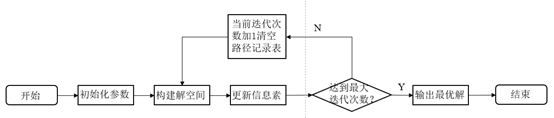图1 蚁群算法解决TSP问题的基本步骤 1：初始化参数 在计算之初，需要对相关的参数进行初始化，如蚁群规模、信息素重要程度因子、启发函数重要程度因子，信息素挥发因子，信息素释放总量，最大迭代次数，迭代数初值。 2：构建解空间 将各个蚂蚁随机地置于不同出发点，对每个蚂蚁k，计算其下一个待访问的城市，直到所有蚂蚁访问完所有的城市。 3：更新信息素 计算各个蚂蚁经过的路径长度，记录当前迭代次数中的最优解（最短路径）。同时，对各个城市连接路径上的信息素浓度进行更新。 4：判断是否终止 若iter<iter_max，则令iter=iter+1，清空蚂蚁经过路径的记录表，并返回步骤2，否则，终止计算，输出最优解。
蚁群算法的特点： （1）采用正反馈机制，使得搜索过程不断收敛，最终逼近最优解。 （2）每个个体可以通过释放信息素来改变周围的环境，且每个个体能够感知周围环境的实时变化，个体间通过环境进行间接地通讯。 （3）搜索过程采用分布式计算方法，多个个体同时进行并行计算，大大提高了算法的计算能力和运行效率。 （4）启发式的概率搜索方法不容易陷入局部最优解，易于寻找到全局最优解。
我们以中国31个直辖市、省会、自治区（未包括我国香港、澳门及台湾）
求解结果如下：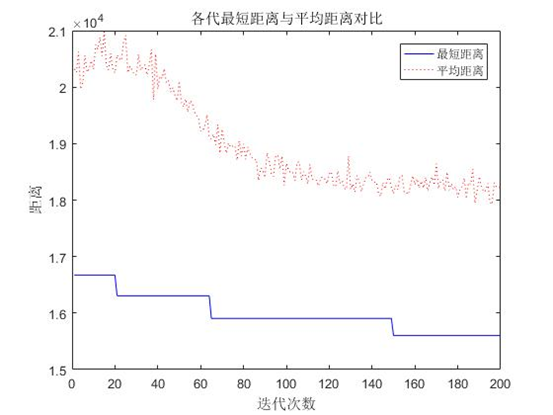图2 TSP的最短距离与迭代次数的关系图图3 蚁群算法优化路径
二维路径规划问题： 路径规划算法是指在有障碍物的工作环境中寻找一条从起点到终点、无碰撞地绕过所有障碍物的运动路径。 我们以一个具体问题为例： 采用蚁群算法在200200的二维空间中寻找一条从起点S到终点T的最优路径，该二维空间中存在4个障碍物，障碍物1的4个顶点的坐标分别为（40 140；60 160；100 140；60 120），障碍物2的4个顶点分别为（50 30；30 40；80 80；100 40），障碍物3的4个顶点分别为（120 160；140 100；180 170；165 180），障碍物4的3个顶点分别为（120 40；170 40；140 80），其中点S为起点，起点坐标为（20,180）；点T为终点，终点坐标为（160,90）。 求解结果：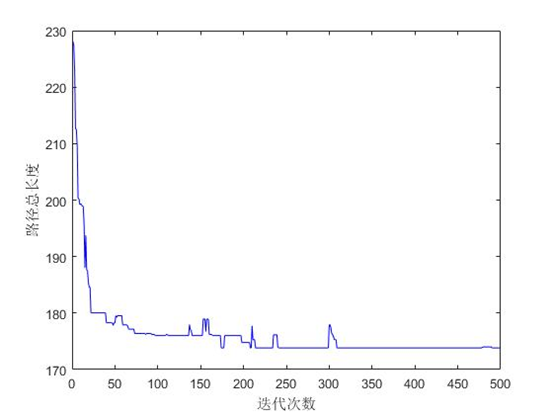图4 适应度值变化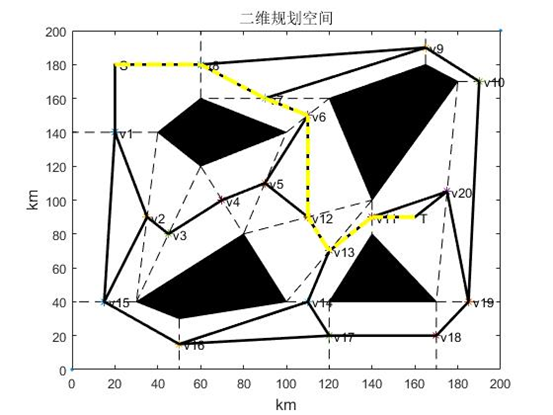图5 路径规划结果 三维路径规划问题： 采用蚁群算法在跨度为21km21km的一片海域中搜索从起点到终点，并且避开所有障碍物的路径，为了方便问题的求解，取该区域内最深点的高度为0，其他点高度根据和最深点高度差依次取得。路径规划起点坐标为（1,10,800），终点坐标为（21,4,1000），规划环境和起点、终点如图：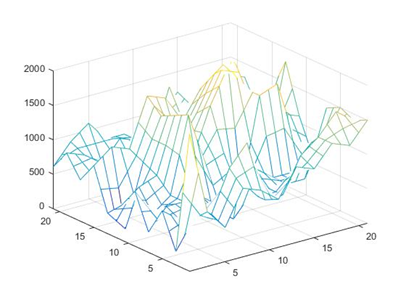图6 三维路径搜索空间 求解结果如图：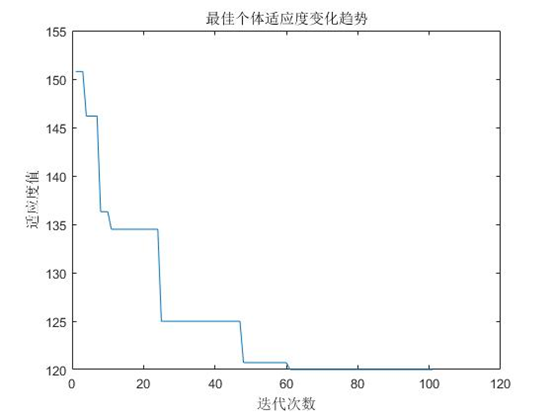图7 适应度值变化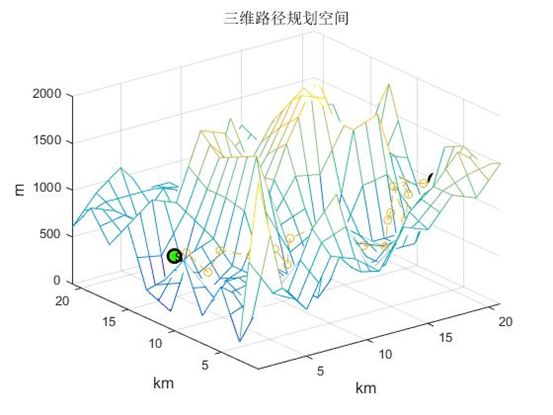图8 路径规划结果 参考文献： DORIGO M,GAMBARDELLA L M. Ant Colonies for the Traveling Salesman Problem[J]. BioSystems,1997,43(2):73-81. DORIGO M,GAMBARDELLA L M Ant Colony System:a Cooperative Learning Approach to the Traveling Salesman Problem[J].IEEE Transaction on Evolutionary Computation,1997,1(1):53-66. DORIGO M,BIRATTARI M,STUTZLE T. Ant Colony Optimization[J].Computational Intelligence Magazine,2006,1(4):28-39. STUTZLE T D M.A Short Convergence Proof for a Class of Ant Colony Optimization Algorithms[J].IEEE Transactions on Evolutionary Computation,2002,6(4):358-365. 萧蕴诗，李炳宇，吴启迪.求解TSP问题的模式学习并行蚁群算法[J].控制与决策，2004,19（8）：885-888. 吴斌，史忠植.一种基于蚁群算法的 TSP问题分段求解方法[J].计算机学报，2001，24(12):1328-1333 王颖，谢剑英.一种自适应蚁群算法及其仿真研究[J].系统仿真学报,2002.14(1):31 -33. 叶志伟，郑肇葆.蚁群算法中参数 设置的研究——以 TSP问题为例[J].武汉大学学报:信息科学版.2004.29(7):597 - 601. 胡小兵，黄席樾.对一类带聚类特征 TSP问题的蚁群算法求解[J].系统仿真学报，2004,16(12)： 2683 - 2686.  徐精明，曹先彬，王煦法.多态蚁群算法[J].中国科学技术大学学报，2005，35(1):59- 65.
XI Y G.ZHANG C G. Rolling Path Planning of Mobile Robot in a Kind of Dynamic Uncertain Environment[J]. Acta Automatica Sinica,2002,28:161 - 175.  COLONIA DORIGO M. MANIEZZO V. Distributed Optimization by Ant Colonies[EB/OL]. [2010 - 09]. ftp://iridia. ulb. ac. be/ pub/ mdorigo/ conference/IC.06 - ECAL92. pdf.  DORIGO M, MANIEZZO V, COLONI A. The Ant System: Optimization by a Colony of Cooperating Agents[ EB/OL]. [2010- 09]. http://ieeexplore.ieee.org/xpl/freeabs_ all. jsp? arnumber=484436.  DORIGO M, GAMBARDELLA L M. Ant Colony System: a Cooperative Learning Approach to the Traveling Salesman Problem[J]. IEEE Transaction on Evolutionary Computation. 1997,1:53 - 66.
张京娟.基于遗传算法的水下潜器自主导航规划技术研究[D].哈尔滨:哈尔滨工程大学,2003.  WARREN C W. A Technique for Autonomous Underwater Vehicle Route Planning[J]. IEEE Journal of Oceanic Engineering, 1990,15(3):199 - 204.  VASUDEVAN C. GANESAN L. Case based Path Planning for Autonomous Underwater Vehicles[J]. Autonomous Robots, 1996,3(2):79 - 89. 田峰敏.基于先验地形数据处理的水下潜器地形辅助导航方法研究[D].哈尔滨:哈尔滨工程大学2007. 附录：matlab代码： TSP问题求解代码：
%% 清空环境变量
clear all
clc

%% 导入数据

%% 计算城市间相互距离
n = size(citys,1);
D = zeros(n,n);
for i = 1:n
for j = 1:n
if i ~= j
D(i,j) = sqrt(sum((citys(i,:) - citys(j,:)).^2));
else
D(i,j) = 1e-4;
end
end
end

%% 初始化参数
m = 50;                              % 蚂蚁数量
alpha = 1;                           % 信息素重要程度因子
beta = 5;                            % 启发函数重要程度因子
rho = 0.1;                           % 信息素挥发因子
Q = 1;                               % 常系数
Eta = 1./D;                          % 启发函数
Tau = ones(n,n);                     % 信息素矩阵
Table = zeros(m,n);                  % 路径记录表
iter = 1;                            % 迭代次数初值
iter_max = 200;                      % 最大迭代次数
Route_best = zeros(iter_max,n);      % 各代最佳路径
Length_best = zeros(iter_max,1);     % 各代最佳路径的长度
Length_ave = zeros(iter_max,1);      % 各代路径的平均长度

%% 迭代寻找最佳路径
while iter <= iter_max
% 随机产生各个蚂蚁的起点城市
start = zeros(m,1);
for i = 1:m
temp = randperm(n);
start(i) = temp(1);
end
Table(:,1) = start;
% 构建解空间
citys_index = 1:n;
% 逐个蚂蚁路径选择
for i = 1:m
% 逐个城市路径选择
for j = 2:n
tabu = Table(i,1:(j - 1));           % 已访问的城市集合(禁忌表)
allow_index = ~ismember(citys_index,tabu);
allow = citys_index(allow_index);  % 待访问的城市集合
P = allow;
% 计算城市间转移概率
for k = 1:length(allow)
P(k) = Tau(tabu(end),allow(k))^alpha * Eta(tabu(end),allow(k))^beta;
end
P = P/sum(P);
% 轮盘赌法选择下一个访问城市
Pc = cumsum(P);
target_index = find(Pc >= rand);
target = allow(target_index(1));
Table(i,j) = target;
end
end
% 计算各个蚂蚁的路径距离
Length = zeros(m,1);
for i = 1:m
Route = Table(i,:);
for j = 1:(n - 1)
Length(i) = Length(i) + D(Route(j),Route(j + 1));
end
Length(i) = Length(i) + D(Route(n),Route(1));
end
% 计算最短路径距离及平均距离
if iter == 1
[min_Length,min_index] = min(Length);
Length_best(iter) = min_Length;
Length_ave(iter) = mean(Length);
Route_best(iter,:) = Table(min_index,:);
else
[min_Length,min_index] = min(Length);
Length_best(iter) = min(Length_best(iter - 1),min_Length);
Length_ave(iter) = mean(Length);
if Length_best(iter) == min_Length
Route_best(iter,:) = Table(min_index,:);
else
Route_best(iter,:) = Route_best((iter-1),:);
end
end
% 更新信息素
Delta_Tau = zeros(n,n);
% 逐个蚂蚁计算
for i = 1:m
% 逐个城市计算
for j = 1:(n - 1)
Delta_Tau(Table(i,j),Table(i,j+1)) = Delta_Tau(Table(i,j),Table(i,j+1)) + Q/Length(i);
end
Delta_Tau(Table(i,n),Table(i,1)) = Delta_Tau(Table(i,n),Table(i,1)) + Q/Length(i);
end
Tau = (1-rho) * Tau + Delta_Tau;
% 迭代次数加1，清空路径记录表
iter = iter + 1;
Table = zeros(m,n);
end

%% 结果显示
[Shortest_Length,index] = min(Length_best);
Shortest_Route = Route_best(index,:);
disp(['最短距离:' num2str(Shortest_Length)]);
disp(['最短路径:' num2str([Shortest_Route Shortest_Route(1)])]);

%% 绘图
figure(1)
plot([citys(Shortest_Route,1);citys(Shortest_Route(1),1)],...
[citys(Shortest_Route,2);citys(Shortest_Route(1),2)],'o-');
grid on
for i = 1:size(citys,1)
text(citys(i,1),citys(i,2),['   ' num2str(i)]);
end
text(citys(Shortest_Route(1),1),citys(Shortest_Route(1),2),'       起点');
text(citys(Shortest_Route(end),1),citys(Shortest_Route(end),2),'       终点');
xlabel('城市位置横坐标')
ylabel('城市位置纵坐标')
title(['蚁群算法优化路径(最短距离:' num2str(Shortest_Length) ')'])
figure(2)
plot(1:iter_max,Length_best,'b',1:iter_max,Length_ave,'r:')
legend('最短距离','平均距离')
xlabel('迭代次数')
ylabel('距离')
title('各代最短距离与平均距离对比')

%%

二维路线规划代码：
%% 清空环境
clc;clear

%% 障碍物数据
plot([0,200],[0,200],'.');
hold on
xlabel('km','fontsize',12)
ylabel('km','fontsize',12)
title('二维规划空间','fontsize',12)
%% 描述起点和终点
S = [20,180];
T = [160,90];
plot([S(1),T(1)],[S(2),T(2)],'.');

% 图形标注
text(S(1)+2,S(2),'S');
text(T(1)+2,T(2),'T');

%% 描绘障碍物图形
fill(position(1:4,1),position(1:4,2),[0,0,0]);
fill(position(5:8,1),position(5:8,2),[0,0,0]);
fill(position(9:12,1),position(9:12,2),[0,0,0]);
fill(position(13:15,1),position(13:15,2),[0,0,0]);

% 下载链路端点数据

%% 描绘线及中点
v = zeros(size(L));
for i=1:20
plot([position(L(i,1),1),position(L(i,2),1)],[position(L(i,1),2)...
,position(L(i,2),2)],'color','black','LineStyle','--');
v(i,:) = (position(L(i,1),:)+position(L(i,2),:))/2;
plot(v(i,1),v(i,2),'*');
text(v(i,1)+2,v(i,2),strcat('v',num2str(i)));
end

%% 描绘可行路径
[n,m]=size(sign);

for i=1:n

if i == 1
for k=1:m-1
if sign(i,k) == 1
plot([S(1),v(k-1,1)],[S(2),v(k-1,2)],'color',...
'black','Linewidth',2,'LineStyle','-');
end
end
continue;
end

for j=2:i
if i == m
if sign(i,j) == 1
plot([T(1),v(j-1,1)],[T(2),v(j-1,2)],'color',...
'black','Linewidth',2,'LineStyle','-');
end
else
if sign(i,j) == 1
plot([v(i-1,1),v(j-1,1)],[v(i-1,2),v(j-1,2)],...
'color','black','Linewidth',2,'LineStyle','-');
end
end
end
end
path = DijkstraPlan(position,sign);
j = path(22);
plot([T(1),v(j-1,1)],[T(2),v(j-1,2)],'color','yellow','LineWidth',3,'LineStyle','-.');
i = path(22);
j = path(i);
count = 0;
while true
plot([v(i-1,1),v(j-1,1)],[v(i-1,2),v(j-1,2)],'color','yellow','LineWidth',3,'LineStyle','-.');
count = count + 1;
i = j;
j = path(i);
if i == 1 || j==1
break;
end
end
plot([S(1),v(i-1,1)],[S(2),v(i-1,2)],'color','yellow','LineWidth',3,'LineStyle','-.');

count = count+3;
pathtemp(count) = 22;
j = 22;
for i=2:count
pathtemp(count-i+1) = path(j);
j = path(j);
end
path = pathtemp;
path = [1     9     8     7    13    14    12    22];

%% 蚁群算法参数初始化
pathCount = length(path)-2;          %经过线段数量
pheCacuPara=2;                       %信息素计算参数
pheThres = 0.8;                      %信息素选择阈值
pheUpPara=[0.1 0.0003];              %信息素更新参数
qfz= zeros(pathCount,10);            %启发值

phePara = ones(pathCount,10)*pheUpPara(2);         %信息素
qfzPara1 = ones(10,1)*0.5;           %启发信息参数
qfzPara2 = 1.1;                      %启发信息参数
m=10;                                %种群数量
NC=500;                              %循环次数
pathk = zeros(pathCount,m);          %搜索结果记录
shortestpath = zeros(1,NC);          %进化过程记录

%% 初始最短路径
dijpathlen = 0;
vv = zeros(22,2);
vv(1,:) = S;
vv(22,:) = T;
vv(2:21,:) = v;
for i=1:pathCount-1
dijpathlen = dijpathlen + sqrt((vv(path(i),1)-vv(path(i+1),1))^2+(vv(path(i),2)-vv(path(i+1),2))^2);
end
LL = dijpathlen;

%% 经过的链接线
lines = zeros(pathCount,4);
for i = 1:pathCount
lines(i,1:2) = B(L(path(i+1)-1,1),:);
lines(i,3:4) = B(L(path(i+1)-1,2),:);
end

%% 循环搜索
for num = 1:NC

%% 蚂蚁迭代寻优一次
for i=1:pathCount
for k=1:m
q = rand();
qfz(i,:) = (qfzPara2-abs((1:10)'/10-qfzPara1))/qfzPara2; %启发信息
if q<=pheThres%选择信息素最大值
arg = phePara(i,:).*(qfz(i,:).^pheCacuPara);
j = find(arg == max(arg));
pathk(i,k) = j(1);
else  % 轮盘赌选择
arg = phePara(i,:).*(qfz(i,:).^pheCacuPara);
sumarg = sum(arg);
qq = (q-pheThres)/(1-pheThres);
qtemp = 0;
j = 1;
while qtemp < qq
qtemp = qtemp + (phePara(i,j)*(qfz(i,j)^pheCacuPara))/sumarg;
j=j+1;
end
j=j-1;
pathk(i,k) = j(1);
end
% 信息素更新
phePara(i,j) = (1-pheUpPara(1))*phePara(i,j)+pheUpPara(1)*pheUpPara(2);
end
end

%% 计算路径长度
len = zeros(1,k);
for k=1:m
Pstart = S;
Pend = lines(1,1:2) + (lines(1,3:4)-lines(1,1:2))*pathk(1,k)/10;
for l=1:pathCount
len(1,k) = len(1,k)+sqrt(sum((Pend-Pstart).^2));
Pstart = Pend;
if l<pathCount
Pend = lines(l+1,1:2) + (lines(l+1,3:4)-lines(l+1,1:2))*pathk(l+1,k)/10;
end
end
Pend = T;
len(1,k) = len(1,k)+sqrt(sum((Pend-Pstart).^2));
end

%% 更新信息素
% 寻找最短路径
minlen = min(len);
minlen = minlen(1);
minant = find(len == minlen);
minant = minant(1);

% 更新全局最短路径
if minlen < LL
LL = minlen;
end

% 更新信息素
for i=1:pathCount
phePara(i,pathk(i,minant)) = (1-pheUpPara(1))* phePara(i,pathk(i,minant))+pheUpPara(1)*(1/minlen);
end
shortestpath(num) = minlen;
end

figure;
plot(1:NC,shortestpath,'color','blue');
hold on
% plot(1:NC,dijpathlen,'color','red');
ylabel('路径总长度');
xlabel('迭代次数');

function path = DijkstraPlan(position,sign)
%% 基于Dijkstra算法的路径规划算法
%position    input     %节点位置
%sign        input     %节点间是否可达

%path        output    %规划路径

%% 计算路径距离
cost = ones(size(sign))*10000;
[n,m] = size(sign);
for i = 1:n
for j = 1:m
if sign(i,j) == 1
cost(i,j) = sqrt(sum((position(i,:)-position(j,:)).^2));
end
end
end

%% 路径开始点
dist = cost(1,:);             %节点间路径长度
s = zeros(size(dist));        %节点经过标志
s(1) = 1;dist(1) = 0;
path = zeros(size(dist));     %依次经过的节点
path(1,:) = 1;

%% 循环寻找路径点
for num = 2:n

% 选择路径长度最小点
mindist = 10000;
for i = 1:length(dist)
if s(i) == 0
if dist(i)< mindist
mindist = dist(i);
u = i;
end
end
end

% 更新点点间路径
s(u) = 1;
for w = 1:length(dist)
if s(i) == 0
if dist(u)+cost(u,w) < dist(w)
dist(w) = dist(u)+cost(u,w);
path(w) = u;
end
end
end
end

三维路线规划代码：
%% 该函数用于演示基于蚁群算法的三维路径规划算法

%% 清空环境
clc
clear

%% 数据初始化

%下载数据

%网格划分
LevelGrid=10;
PortGrid=21;

%起点终点网格点
starty=10;starth=4;
endy=8;endh=5;
m=1;
%算法参数
PopNumber=10;         %种群个数
BestFitness=[];    %最佳个体

%初始信息素
pheromone=ones(21,21,21);

%% 初始搜索路径
[path,pheromone]=searchpath(PopNumber,LevelGrid,PortGrid,pheromone, ...
HeightData,starty,starth,endy,endh);
fitness=CacuFit(path);                          %适应度计算
[bestfitness,bestindex]=min(fitness);           %最佳适应度
bestpath=path(bestindex,:);                     %最佳路径
BestFitness=[BestFitness;bestfitness];          %适应度值记录

%% 信息素更新
rou=0.2;
cfit=100/bestfitness;
for i=2:PortGrid-1
pheromone(i,bestpath(i*2-1),bestpath(i*2))= ...
(1-rou)*pheromone(i,bestpath(i*2-1),bestpath(i*2))+rou*cfit;
end

%% 循环寻找最优路径
for kk=1:100

%% 路径搜索
[path,pheromone]=searchpath(PopNumber,LevelGrid,PortGrid,...
pheromone,HeightData,starty,starth,endy,endh);

%% 适应度值计算更新
fitness=CacuFit(path);
[newbestfitness,newbestindex]=min(fitness);
if newbestfitness<bestfitness
bestfitness=newbestfitness;
bestpath=path(newbestindex,:);
end
BestFitness=[BestFitness;bestfitness];

%% 更新信息素
cfit=100/bestfitness;
for i=2:PortGrid-1
pheromone(i,bestpath(i*2-1),bestpath(i*2))=(1-rou)* ...
pheromone(i,bestpath(i*2-1),bestpath(i*2))+rou*cfit;
end

end

%% 最佳路径
for i=1:21
a(i,1)=bestpath(i*2-1);
a(i,2)=bestpath(i*2);
end
figure(1)
x=1:21;
y=1:21;
[x1,y1]=meshgrid(x,y);
mesh(x1,y1,HeightData)
axis([1,21,1,21,0,2000])
hold on
k=1:21;
plot3(k(1)',a(1,1)',a(1,2)'*200,'--o','LineWidth',2,...
'MarkerEdgeColor','k',...
'MarkerFaceColor','g',...
'MarkerSize',10)
plot3(k(21)',a(21,1)',a(21,2)'*200,'--o','LineWidth',2,...
'MarkerEdgeColor','k',...
'MarkerFaceColor','g',...
'MarkerSize',10)
text(k(1)',a(1,1)',a(1,2)'*200,'S');
text(k(21)',a(21,1)',a(21,2)'*200,'T');
xlabel('km','fontsize',12);
ylabel('km','fontsize',12);
zlabel('m','fontsize',12);
title('三维路径规划空间','fontsize',12)
set(gcf, 'Renderer', 'ZBuffer')
hold on
plot3(k',a(:,1)',a(:,2)'*200,'--o')

%% 适应度变化
figure(2)
plot(BestFitness)
title('最佳个体适应度变化趋势')
xlabel('迭代次数')
ylabel('适应度值')

function fitness=CacuFit(path)
%% 该函数用于计算个体适应度值
%path       input     路径
%fitness    input     路径

[n,m]=size(path);
for i=1:n
fitness(i)=0;
for j=2:m/2
%适应度值为长度加高度
fitness(i)=fitness(i)+sqrt(1+(path(i,j*2-1)-path(i,(j-1)*2-1))^2 ...
+(path(i,j*2)-path(i,(j-1)*2))^2)+abs(path(i,j*2));
end
End

function qfz=CacuQfz(Nexty,Nexth,Nowy,Nowh,endy,endh,abscissa,HeightData)
%% 该函数用于计算各点的启发值
%Nexty Nexth    input    下个点坐标
%Nowy Nowh      input    当前点坐标
%endy endh      input    终点坐标
%abscissa       input    横坐标
%HeightData     input    地图高度
%qfz            output   启发值

%% 判断下个点是否可达
if HeightData(Nexty,abscissa)<Nexth*200
S=1;
else
S=0;
end

%% 计算启发值
%D距离
D=50/(sqrt(1+(Nowh*0.2-Nexth*0.2)^2+(Nexty-Nowy)^2)+sqrt((21-abscissa)^2 ...
+(endh*0.2-Nexth*0.2)^2+(endy-Nowy)^2));
%计算高度
M=30/abs(Nexth+1);
%计算启发值
qfz=S*M*D;

function [path,pheromone]=searchpath(PopNumber,LevelGrid,PortGrid,pheromone,HeightData,starty,starth,endy,endh)
%% 该函数用于蚂蚁蚁群算法的路径规划
%LevelGrid     input    横向划分格数
%PortGrid      input    纵向划分个数
%pheromone     input    信息素
%HeightData    input    地图高度
%starty starth input    开始点
%path          output   规划路径
%pheromone     output   信息素

%% 搜索参数
ycMax=2;   %蚂蚁横向最大变动
hcMax=2;   %蚂蚁纵向最大变动
decr=0.9;  %信息素衰减概率

%% 循环搜索路径
for ii=1:PopNumber

path(ii,1:2)=[starty,starth];  %记录路径
NowPoint=[starty,starth];      %当前坐标点

%% 计算点适应度值
for abscissa=2:PortGrid-1
%计算所有数据点对应的适应度值
kk=1;
for i=-ycMax:ycMax
for j=-hcMax:hcMax
NextPoint(kk,:)=[NowPoint(1)+i,NowPoint(2)+j];
if (NextPoint(kk,1)<20)&&(NextPoint(kk,1)>0)&&(NextPoint(kk,2)<20)&&(NextPoint(kk,2)>0)
qfz(kk)=CacuQfz(NextPoint(kk,1),NextPoint(kk,2),NowPoint(1),NowPoint(2),endy,endh,abscissa,HeightData);
qz(kk)=qfz(kk)*pheromone(abscissa,NextPoint(kk,1),NextPoint(kk,2));
kk=kk+1;
else
qz(kk)=0;
kk=kk+1;
end
end
end

%选择下个点
sumq=qz./sum(qz);

pick=rand;
while pick==0
pick=rand;
end

for i=1:25
pick=pick-sumq(i);
if pick<=0
index=i;
break;
end
end

oldpoint=NextPoint(index,:);

%更新信息素
pheromone(abscissa+1,oldpoint(1),oldpoint(2))=0.5*pheromone(abscissa+1,oldpoint(1),oldpoint(2));

%路径保存
path(ii,abscissa*2-1:abscissa*2)=[oldpoint(1),oldpoint(2)];
NowPoint=oldpoint;

end
path(ii,41:42)=[endy,endh];
end

展开全文蚁群算法 数学建模
• ## Matlab文本生成矩阵

千次阅读 2012-10-19 11:23:46
有时候我们得到数据之后，...假设数据存储在文本中，如何通过Matlab进行处理呢？ 方法参见链接内容：http://wiki.jxqc.org/mediawiki/index.php?title=%E6%96%87%E6%9C%AC%E7%94%9F%E6%88%90%E7%9F%A9%E9%98%B5
有时候我们得到数据之后，需要利用Matlab来进行数据分析和处理。

假设数据存储在文本中，如何通过Matlab进行处理呢？

方法参见链接内容：http://wiki.jxqc.org/mediawiki/index.php?title=%E6%96%87%E6%9C%AC%E7%94%9F%E6%88%90%E7%9F%A9%E9%98%B5
展开全文数据分析 存储 c
• matlab2016a版工具下载链接，64位，此为文本文件，里面是下载链接
• ## MATLAB 2018b 安装与简介

万次阅读 多人点赞 2019-02-18 13:07:00
matlab2018b安装教程 该版本是mathworks官方开发的新版本的商业数学软件，可以帮助用户不仅仅将自己的创意停留在桌面，还可以对大型数据集运行分析，并扩展到群集和云。另外matlab代码可以与其他语言集成，使您...
matlab2018b、2019a安装教程

下载地址：
由于2018版本安装包丢失，现附上2019A版的下载链接，破解过程类似。
请复制链接及提取码并打开，直接点击链接会被屏蔽
链接：https://pan.baidu.com/s/1kENZPKNIpni3taiy-jE4ng  zwm3
另附2018b版本下载链接：
链接：https://pan.baidu.com/s/1n3feN1SUwbAIW19AePr6bg 提取码：b590
感谢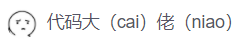同学维护并分享资源！matlab2018b破解版安装教程：
1、下载解压，得到mathworks matlab r2018b中文原程序和破解文件。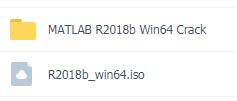2、首先用winrar解压“R2018b_win64.iso”文件，或者使用虚拟光驱加载，运行里面的“setup.exe”进行原版程序安装，首先选择使用文件安装秘钥选项，如下图所示：3、阅读并同意软件安装协议。4、在秘钥对话框中输入matlab2018b安装密钥【09806-07443-53955-64350-21751-41297】5、软件默认安装在C盘，因软件占用磁盘空间12G+，建议点击【浏览】更改软件安装位置。6、选择需要安装的产品后，点击下一步，建议全选。7、创建桌面快捷方式和开始菜单。8、确定安装信息。9、等待matlab2018b软件安装完成。10、注意：先不要启动软件，将破解补丁“netapi32.dll”复制到相对应的文件夹中 默认路径为：C:\Program Files\MATLAB\R2018b\bin\win64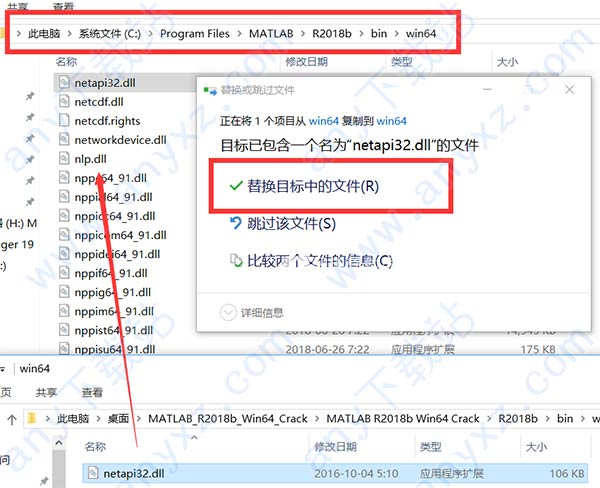11、现在启动软件，然后选择手动激活：12、许可证选择补丁文件夹下的“license_standalone.lic”。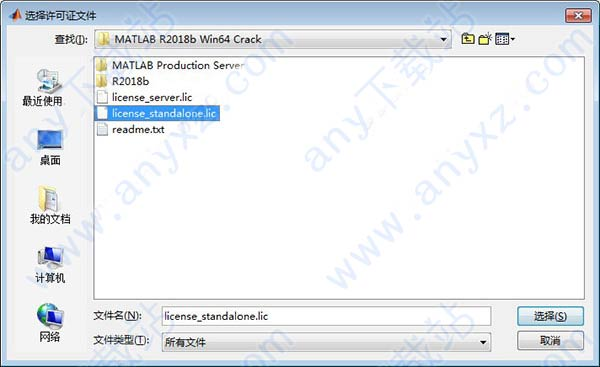13、提示激活完成。14、至此，matlab2018b破解版完成激活，用户可以无限制免费使用。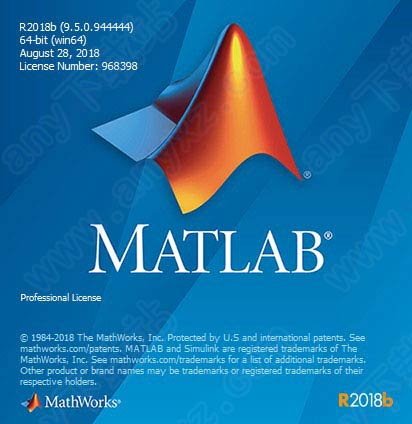matlab2018b新功能：
一、实时编辑器 所创建的脚本不仅可以捕获代码，还可以讲述与人分享的故事。自动化的上下文提示可让您在编程时快速推进，并且将结果与可视化内容和您的代码一起显示。       二、App Designer App Designer 让您无需成为专业的软件开发人员，即可创建专业的应用程序。拖放可视化组件来安排应用程序布局，并使用集成编辑器快速编写应用程序的行为。您可以使用 MATLAB Compiler 创建独立的桌面或 Web 应用程序以共享您的应用程序。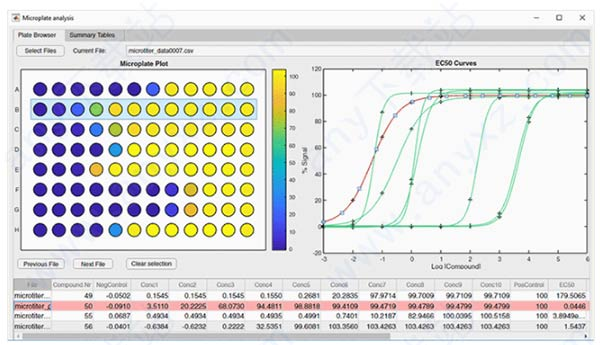三、数据分析 您有了数据，不要将所有时间花费在为分析准备数据上。使用 MATLAB 中的新工具和功能来导入、清理、筛选和分组数据，并更快地开始分析。       四、大数据 无需学习大数据编程 — MATLAB 中的 tall 数组可让您使用惯用的代码和语法，即使您的数据集无法装入内存。MATLAB 支持您已在使用的存储系统，包括传统文件系统、SQL 和 NoSQL 数据库以及 Hadoop/HDFS。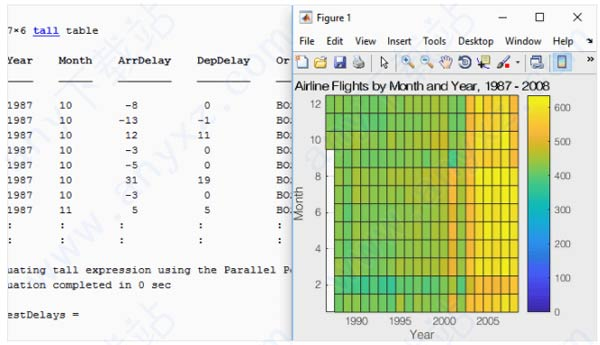五、性能 matlab2018b运行代码的速度几乎是两年前的两倍。而且不需要对您的代码做出任何更改。     六、图形 MATLAB 图形系统使创建和自定义绘图变得简单，且新的默认颜色、字体和样式使您的数据更容易解读。浏览新绘图，包括： 1、geobubble 2、wordcloud 3、heatmap 4、polarplot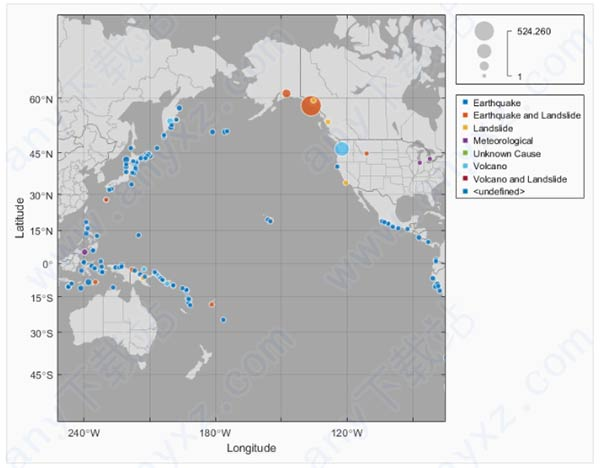七、团队开发 随着项目规模和复杂程度的增长，MATLAB 提供了支持协作软件开发实践的能力。 1、面向对象编程 2、源代码管理集成 3、测试框架 4、与 C/C++、Java、.NET 和 Python 集成八、硬件支持 matlab2018b可以控制诸如 Arduino 和 Raspberry Pi 这样的流行微控制器，采集网络摄像头中的图像，甚至可以收集智能手机内置传感器中的数据。浏览受支持的硬件： 1、Arduino 2、Raspberry Pi 3、USB 网络摄像头 4、iPhone、iPad 和 Android 设备 5、ThingSpeak IoT九、数据分析 利用matlab2018b，不管您正在处理的是商业数据还是工程数据，您都可以自己做数据科学。无论是部署在传统 IT 平台上应用程序还是在嵌入式平台上应用程序，您都可以随时随地运行分析。十、深度学习 matlab2018b使每个人都能进行深度学习，即使非专业人士也不例外。设计您自己的模型、访问最新模型或导入来自 Caffe 的预训练模型。使用 NVIDIA GPU 训练模型。自动生成嵌入式部署的 CUDA 代码。mathworks matlab r2018b更新日志
一、桌面
实时编辑器：使用其他子标题样式组织实时脚本 实时编辑器：使用内部超链接在实时脚本中导航 实时编辑器：以交互方式过滤表格输出，然后将生成的代码添加到实时脚本中 实时编辑器：更快地创建新的和打开现有的实时脚本 实时编辑器：更改文本或代码的大小写 比较工具：合并两个版本的实时脚本或函数 加载项管理器：安装和管理自定义工具箱的多个版本 加载项管理器：将加载项保存到新的默认位置 文档：以西班牙语查看MATLAB文档 工具箱打包：使用自定义工具箱安装其他软件
二、语言和编程
string数组：string在MATLAB，Simulink和Stateflow中使用数组 convertContainedStringsToChars 功能：在单元格数组或结构的任何级别转换字符串数组 枚举：使用枚举提高集合操作的性能 WSDL Web服务文档：必需的工具更新 功能被删除或更改
三、数学
boundaryshape功能：polyshape 从二维三角测量创建一个对象 polyshape 对象：指定在创建时保持共线点的时间 polyshape RandStream 对象：使用Threefry和Philox算法生成随机数 GraphPlot 对象：使用字体属性自定义节点和边标签 sinpi和cospi函数：计算π倍数的正弦和余弦
四、图像
轴交互：默认情况下启用平移，缩放，数据提示和三维旋转来探索数据 轴工具栏：访问和自定义每个Axes对象的数据探索工具栏 地理图：在交互式地图上创建线，散点图和点密度图，并控制地理轴的属性 stackedplot功能：绘制表或时间表的变量，以便使用通用x轴进行比较 scatterhistogram 功能：将分组数据可视化为具有边缘直方图的散点图 sgtitle 功能：为子图网格创建标题 xline和yline功能：向绘图添加垂直或水平线 imtile 功能：将多个图像帧合并为一个矩形平铺图像 数据提示：在数据提示中使用TeX或LaTeX标记，改善视觉外观 功能被删除或更改
五、数据导入和导出
六、数据分析
矢量尺寸参数：一次操作多个尺寸，用于选定的缩小功能 grouptransform 功能：按组转换表或时间表数据 groupsummary 功能：对矩阵执行组摘要计算 tall 数组：编写自定义算法以在高数组上运行 tall阵列：在高层阵列操作具有更多的功能，包括conv2，wordcloud和 groupsummary rmoutliers 功能：删除数组，表或时间表中的异常值 islocalmin和islocalmax功能：指定突出计算的数据范围 表和时间表元数据：存储每个变量的自定义元数据 timetable 数据类型：以常规时间步长存储行时间时节省内存 timerange 功能：指定定义时间范围的时间单位 convertvars 功能：将表或时间表变量转换为指定的数据类型 table，timetable和 addvars函数：对输入名称使用单引号，而不是双引号字符串 功能被删除或更改
七、App Building
App Designer：在App Designer画布上添加和配置日期选择组件 App Designer：设计视图和代码视图中的统一属性检查器 应用程序设计器：展开和折叠代码视图中的代码部分 App Designer：将应用程序导出为代码文件 应用程序设计器：使用代码分析器消息栏在代码中查找错误和警告 App Designer：使用改进的代码建议和完成程序更快地编写应用程序 App Designer：使用MATLAB首选项控制App Designer代码视图设置 uigridlayout 功能：使用网格布局管理器配置应用布局 滚动容器：启用滚动图形，面板，选项卡和按钮组容器 图交互：使用使用该uifigure函数创建的图形，使用自定义鼠标和键盘交互创建应用程序 显卡支持：使用集成地块成一个应用程序axes， polaraxes以及geoaxes功能 工具提示：为应用中的UI组件创建自定义工具提示 部署的Web应用程序：使用uigetfile和uiputfile功能访问已部署的Web应用程序中的文件 在浏览器中运行应用程序：使用大多数现代浏览器在MATLAB Online或部署的Web应用程序中运行应用 程序 uisetcolor 功能：以交互方式选择自定义颜色 功能被删除或更改
八、性能
启动：提高MATLAB启动速度 执行引擎：在使用冒号运算符时索引到具有改进性能的大型数组 执行引擎：更快地调用内置函数 实时编辑器：更快地创建新的和打开现有的实时脚本 枚举：使用枚举改进集函数性能 构建应用程序：App Designer中更快的画布交互 运行应用程序：更快的应用程序启动时间 sort 功能：更快地对矩阵和数组进行排序
九、硬件支持
MATLAB 在线：沟通与树莓派从硬件板 MATLAB 在线 在Raspberry Pi硬件上部署MATLAB函数 iOS和Android传感器：当您的设备无法访问网络时获取传感器数据 iOS和Android传感器：将传感器日志从设备上传到MATLAB Drive
十、高级软件开发
选项卡完成：使用validateFunctionSignaturesJSON函数验证函数签名文件 选项卡完成：用于functionSignatures.json 升级的JSON解析器 Java SE 8：MATLAB支持，提供更高的安全性和对新Java功能的访问 Python接口：在MATLAB和Python之间传递多维数字或逻辑数组 C ++ MEX API：使用C ++ API从MEX文件中异步调用MATLAB 单元测试框架：与更多插件和更智能的调度并行运行测试 单元测试框架：在参数化测试中使用外部参数 单元测试框架：基于共享夹具对测试套件进行排序 单元测试框架：显式控制输出显示细节和记录的诊断级别 单元测试框架：配置输出诊断的详细级别 单元测试框架：使用约束时更快地比较值 应用程序测试框架：以编程方式选择树节点 性能测试框架：使用该TestCase.keepMeasuring方法更准确地测量快速代码的执行时间 模拟框架：在模拟方法调用时调用函数 模拟框架：按顺序验证模拟上的交互 模拟框架：记录模拟对象交互的清晰历史 matlab.test.behavior.Missing class：验证类满足缺失值行为合同 MEX函数：使用Interleaved Complex API构建Fortran MEX文件 编译器支持已更改，用于构建MEX文件和独立的MATLAB引擎和MAT文件应用程序 系统对象：调用System对象时对输入的灵活要求 系统对象创作：使用枚举在System对象中定义有限属性列表 参考架构：在Amazon Web Services（AWS）和Microsoft Azure上部署和运行MATLAB Git Stashes：存储未提交的更改以供以后使用 功能被删除或更改
展开全文• matlab文件读取总结matlab的文件读取函数功能可以读取各种文件类型
matlab文件读取总结
matlab的文件读取函数功能可以读取各种文件类型，包括

[column1，column2,...] = textread('filename'，'%s %d ...','headlines',number,'delimiter',',','emptyvalue',NAN);
[column1，column2,...] = textread('filename'，'point%d%d...','headlines',number,'delimiter',',','emptyvalue',NAN);
的格式，就会忽略第一个字段中的字符类型。  还有一个比较有意思的用法涉及到正则表达式的使用。例如：  如果只想读第一列，其余的跳过
filename = '.\myfile.txt';

%[^...] - reads characters not matching characters between the brackets until first matching character
%[^\n] 就是一直读到行尾。
%*[^\n] 就是从当前直接跳到行尾。
可对比
[names，rest]=textread(filename,'%s%[^\n]') 
A = importdata(filename, delimiter, nheaderlines);
A = xlsread('data.xlsx','Sheet1');
A=dlmread('lcode.dat','delimeter')
textscan  使用textscan之前必须要先fopen文件，基本使用示例为
fid = fopen('filename.txt');
C = textscan(fid, ' \%f\; \%f', 'delimiter', ',','EmptyValue', -Inf);
fclose(fid);
matlab对文件中混乱的文本格式读取操作总结
对于本文字符数字较为混乱的文本进行文本操作需要对文件内部进行操作，包括文本位置指针，文件逐行读取，字符匹配等等复杂操作。主要用到的几个函数有

feek(设置指针位置)  ftell(获得指针位置)  frewind(重设指针到文件起始位置)  feof(测试指针是否在文件结束位置)

具体用法：  fseek(fid,-1,’eof’),指针到末尾  fseek(fid,2,’bof’),起始位置后第二个字符位置  ftell(fid);找到指针位置  frewind(fid),指针回到起始  feof(fid)判断指针是否在末尾
最详细的指导资料建议参考matlab的help文档。  参考文献：  1. http://blog.csdn.net/holybin/article/details/44199909  2. https://wenku.baidu.com/view/b3268f154431b90d6c85c745.html  3. http://blog.csdn.net/zm1_1zm/article/details/53341106
展开全文数据 文件读取
• MATLAB-Appdesigner中的文本区域输出方式 首先创建文本区域，从左侧组件库将“文本区域”拖拽到设计视图中，该视图在组件浏览器里的名称为“DIstanceTextArea”， 然后首先建立一个回调函数，假设在计算过程中出现一...
• 深度学习matlab工具箱deeplearningtoolbox-新建文本文档 .txt 深度学习matlab工具箱 deep learning toolbox还没研究过，有研究的，麻烦分享一下心得。 不让上传过大附件，也不能直接给链接，只能这样了
• 另外，需要声明的是，我所使用的MATLAB版本——MATLAB R2017a。 目录 一、使用MATLAB的GUIDE开发环境，来快速创建GUI界面 二、修改MATLAB GUI的.fig和.m文件名 三、修改MATLAB GUI界面的名字 四...
• 文本_提取 这个项目是通过MATLAB使用神经网络从图像中提取字母 先决条件 要运行此代码，您将需要安装了深度学习工具箱的 MATLAB 2018 副本。 了解 VGG-16 的架构和算法以及迁移学习的概念也很有用。 安装 Matlab ...
• 需要提取590行的最后一个数值，用作分析建模作用，matlab实现代码如下。 1. 提取该行的数据保存为文字数组 name = textFileName; fidin = fopen(name,'r'); nline = 0; while ~feof(fidin) tline = fgetl(fidin)...数值
• MATLAB智能算法30个案例分析》是2011年由北京航空航天大学出版社出版的图书，作者是郁磊、史峰、王辉、胡斐… 《MATLAB智能算法30个案例分析》是作者多年从事算法研究的经验总结。讲解了遗传算法、免疫算法、退火...智能优化算法 优化
• 为指向帮助页面文档的超链接提供 HREF 文本从当前在 MATLAB 帮助浏览器 (doc) 中打开的页面获取文档链接。 HTML 文本可以在 MATLAB 帮助页面中提供函数。 当需要参考 MATLAB 文档中的特定帮助页面时，这很有用。 doc...
• — 创建内部页面链接以跳转到页面的其他部分。 — 插入换行符。 —插入指定像素高度的间距。 — 指定任何文本的大小。 — 为任何文本指定颜色。 — 插入您自己的 CSS 类以随意设置文本样式。 — 或者，...
• matlab生成文本乱码You probably already know about Lorem Ipsum or perhaps tools such as Random Text Generator, however what can end up happening is – despite the nature of the text – people still ...
• 题目：matlab解析HEX386文本格式并自动绘图 目录 题目：matlab解析HEX386文本格式并自动绘图 参考链接： https://blog.csdn.net/linzhe_deep/article/details/105510170?spm=1001.2014.3001.5501 一、目的： 二...
• 转载文章，原文链接Matlab中的静态文本框中显示多行内容 有时候，我们在GUI中利用静态文本框显示程序的结果，但是结果很长，一行未必可以显示的开，而静态文本框不像edit或listbox那样通过滚动条来显示多行内容，...
• 靜态文本 链接控件 背景透明的静态文本 文章关联： http://blog.csdn.net/shfhere/archive/2010/04/12/5475303.aspx
• 首先我们通过题目理解知道我们的目的是使用matlab统计出txt文本当中每个汉字的个数,我将其分为三个部分来完成。 1）读取txt文件，去除统计结果当中包含的标点符号 读取txt文档的方式有很多，最后我选择了使用...
• （注：当用‘a’时，如果文本中已经存在数据，不会清空数据，而是在数据之后写入，而‘w’会清空原本的数据，重新写入） 一，如果要保存单行、单列数据 fid=fopen(['d:\','A.txt'],'w');%写入文件路径 for jj...
• MATLAB下复现CVPR 2017 EMOTIC情景情感识别的实验，需要预处理数据和额外下载数据集。 链接：http://sunai.uoc.edu/emotic/?tdsourcetag=s_pctim_aiomsg
• 转载自：https://blog.csdn.net/qq_40969467/article/details/82735611 感谢博主：PtaQ 以下是原博客： matalb中打开文件： fid = fopen(文件名，... 参考链接：https://blog.csdn.net/dmfylb/article/details/72581406
• 文本和字符 字符串数组中的文本 当您处理文本时，将字符序列括在双引号中。可以将文本赋给变量。语句最后加；虽然保存了变量但是不会进行输出。不加；即保存又输出。 例 t = "Hello, world"; 如果文本包含双...
• 实际上，自动LaTeX导出的形式与Matlab语言语法突出显示的形式不匹配，并且不能通过显示特殊字符（例如，注释或文本字符串中的特殊字母或符号）解决问题。 改进在于修改 matlab.sty： - 添加所需的包 matlab-...
• ## matlab匿名函数

千次阅读 2019-01-19 21:09:08
可以将函数句柄及其相关值存储在 MAT 文件中，然后使用 save 和 load 函数在后续的 MATLAB 会话中加载它们，例如 save myfile.mat parabola 在构造匿名函数时仅使用显式变量。如果匿名函数访问未在参数列表或主体...
• spearman的matlab代码神经文本实体编码器 (NTEE) 介绍 神经文本实体编码器 (NTEE) 是一种神经网络模型，可以学习文本和维基百科实体的嵌入（或分布式表示）。 我们的模型将文本及其相关实体放在一个连续的向量空间中...
• 一共三个压缩文件，一个文本文件，两个为源文件，一个为破解软件，文本文件为安装教程，由于软件压缩包很大，这里给出百度网盘链接...

# matlab文本链接matlab 订阅